## Cubes and Dices

#### Cubes and Dices

1. How many cubes/blocks are there in below figure?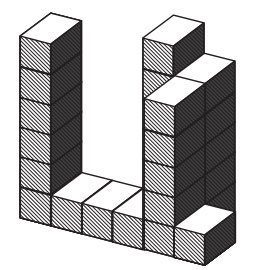1. Total cubes 25

##### Correct Option: B

Total cubes 25

1. How many cubes/blocks are there in below figure?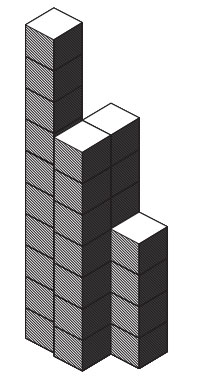1. One column with 10 boxes = 1 × 10 = 10
Two column with 7 boxes = 2 × 7 = 14

##### Correct Option: C

One column with 10 boxes = 1 × 10 = 10
Two column with 7 boxes = 2 × 7 = 14
One column with 4 boxes= 1 × 4 = 4
Total boxes = 28

1. How many cubes/blocks are there in below figure?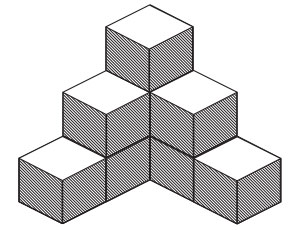1. Total cubes = 9

##### Correct Option: B

Total cubes = 9

1. How many cubes/blocks are there in below figure?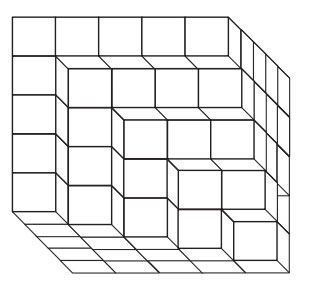1. There are 9 columns with 5 cubes = 9 × 5
There are 7 columns with 4 cubes = 7 × 4
There are 5 columns with 3 cubes = 5 × 3
There are 3 columns with 2 cubes = 3 × 2
There is one column with 1 cubes = 1 × 1

##### Correct Option: C

There are 9 columns with 5 cubes = 9 × 5
There are 7 columns with 4 cubes = 7 × 4
There are 5 columns with 3 cubes = 5 × 3
There are 3 columns with 2 cubes = 3 × 2
There is one column with 1 cubes = 1 × 1
Total = 9 × 5 + 7 × 4 + 5 × 3 + 3 × 2 + 1 × 1 = 81

1. Two positions of a block are shown below (i) and (ii). When 2 dots is at the bottom which number of dots will be at the top?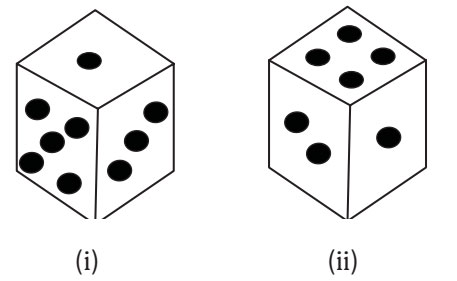1. Number 1 is common to both the figures (i) and (ii). The dice in fig. (ii) is assumed to be rotated so that 1 dot moves to the top face i.e to the same position as in figure (i) and 2 and 4 dots move to the face behind the faces with 3 and 5 dots respectively.

For the better understanding take a dice and apply the same steps which is mentioned above.

##### Correct Option: A

Number 1 is common to both the figures (i) and (ii). The dice in fig. (ii) is assumed to be rotated so that 1 dot moves to the top face i.e to the same position as in figure (i) and 2 and 4 dots move to the face behind the faces with 3 and 5 dots respectively. Clearly by combination, 3 dots lie on the face opposite the face having 2 dots. Therefore, when there are 2 dots at the bottom, the number of dots at the top will be 3.